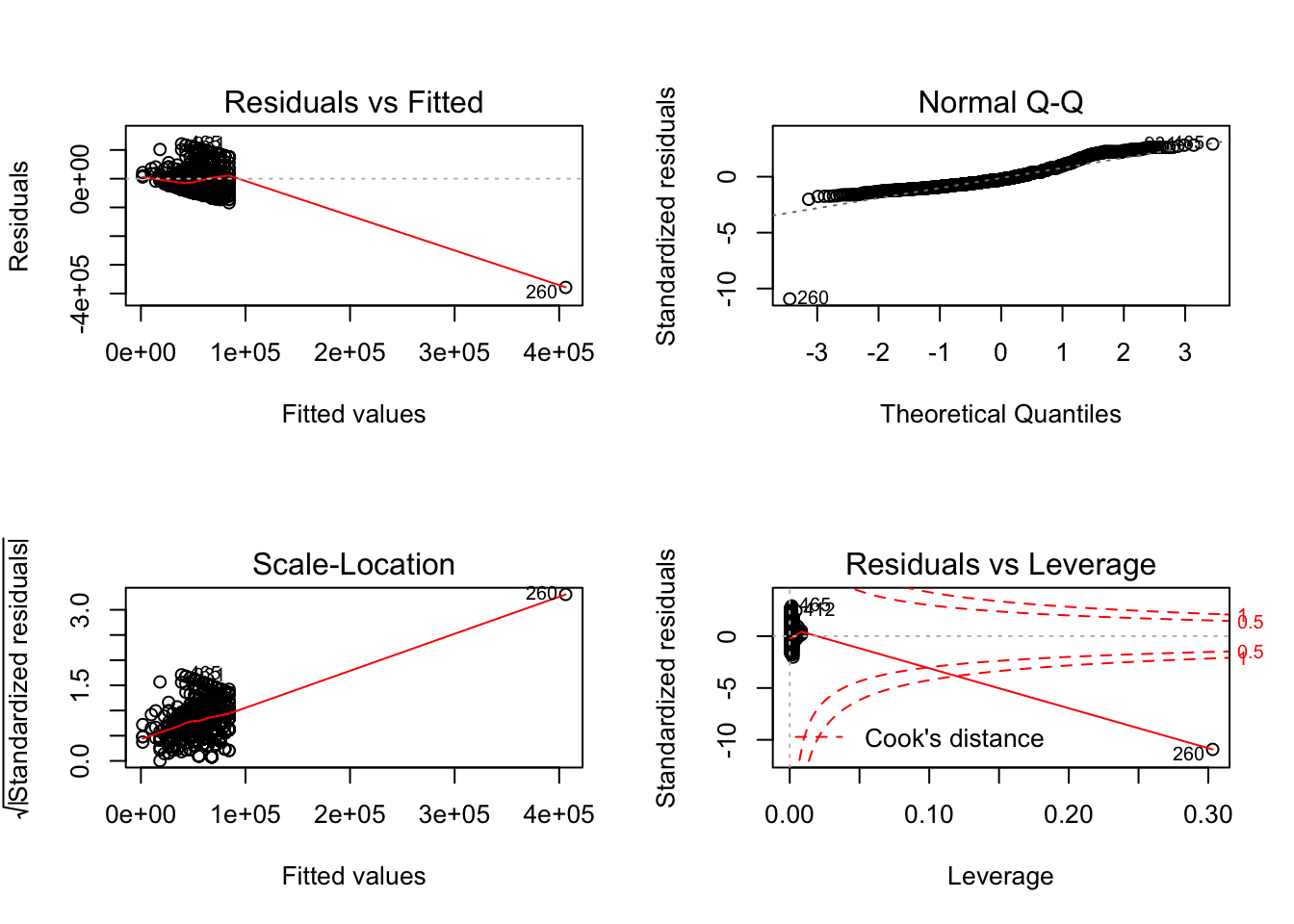## Introduction

Chapter 2 introduces simple linear regression. The following examples help illustrate a number of principles that were discussed. Follow all instructions to complete Chapter 2.

## Simple Regression

1. Download the GSS_reduced_example.csv data set from Canvas or tysonbarrett.com/teaching to your computer. Save it in a directory you can access fairly easily.
2. Open RStudio, start a new R script or RMarkdown document.
3. Load the tidyverse package (you can ignore the notes that you see below that it gives you once you load it).
library(tidyverse)
## ── Attaching packages ───────────────────────────────────────────────────────────────────────── tidyverse 1.2.1.9000 ──
## ✔ ggplot2 2.2.1.9000     ✔ purrr   0.2.5
## ✔ tibble  1.4.2          ✔ dplyr   0.7.5
## ✔ tidyr   0.8.1          ✔ stringr 1.3.1
## ✔ readr   1.1.1          ✔ forcats 0.3.0
## ── Conflicts ───────────────────────────────────────────────────────────────────────────────── tidyverse_conflicts() ──
## ✖ dplyr::lag()    masks stats::lag()
1. Import it into R.
gss <- read.csv("GSS_reduced_example.csv")
1. Use a simple regression model to assess the effect of the number of years of education (educ) on income (income06).
gss %>%
lm(income06 ~ educ,
data = .)
##
## Call:
## lm(formula = income06 ~ educ, data = .)
##
## Coefficients:
## (Intercept)         educ
##        1742         4127
1. This output gives you two values, that for the intercept and that for the slope of educ. What does the slope of educ mean here (i.e., interpret the value)?

## Regression and Correlation

Simple regression and correlation are intimately tied. Let’s show that below.

1. Using the GSS data you already imported above, let’s look at the correlation between income and years of education.
gss %>%
furniture::tableC(income06, educ,
na.rm = TRUE)
## N = 1774
## Note: pearson correlation (p-value).
##
## ─────────────────────────────────
##                         
##  income06 1.00
##  educ     0.341 (<.001) 1.00
## ─────────────────────────────────
1. Let’s compare this to the regression value after we standardize both variables with scale(). We first have to grab just the complete cases of the variables first (consider why).
gss %>%
filter(complete.cases(income06, educ)) %>%
mutate(incomeZ = scale(income06) %>% as.numeric,
educZ = scale(educ) %>% as.numeric) %>%
lm(incomeZ ~ educZ,
data = .)
##
## Call:
## lm(formula = incomeZ ~ educZ, data = .)
##
## Coefficients:
## (Intercept)        educZ
##  -2.466e-16    3.409e-01
1. The intercept is essentially zero and the slope is the same as the correlation before. Is this surprising? Why or why not?

## Residuals

Residuals can help us understand several things about the model and the relations we are assessing. Most of this stuff we’ll talk about later but here’s some ways we’ll access the residuals.

1. Assign the first model to fit (or any other name you want to use).
fit <- gss %>%
lm(income06 ~ educ,
data = .)
1. With that object, use the function resid() to produce the residuals of the model. (Note that head() was used just to see the first 6 lines instead of all two thousand lines.)
resid(fit) %>%
head()
##          1          2          3          4          5         11
## -43763.331  35722.413 -10390.113  -5509.767 -18763.331  88102.759
1. Often we are going to be looking at the residuals in plots. To do this simply, we’ll use plot(). You don’t need to know what the four plots mean, but know that it uses the residuals from the model to do it.
plot(fit)## Conclusion

This was an introduction to many of the features of regression that we’ll be using throughout the class. Although not much of a workflow here, each piece will play a role in larger analyses.